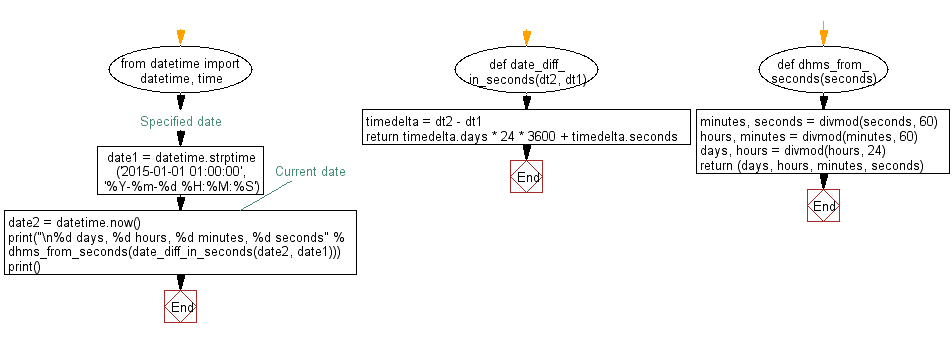﻿ Python: Convert two date difference in days, hours, minutes, seconds - w3resource

# Python: Convert two date difference in days, hours, minutes, seconds

## Python Datetime: Exercise-37 with Solution

Write a Python program to convert difference of two dates into days, hours, minutes, and seconds.

Sample Solution:

Python Code:

``````from datetime import datetime, time

def date_diff_in_seconds(dt2, dt1):
timedelta = dt2 - dt1
return timedelta.days * 24 * 3600 + timedelta.seconds

def dhms_from_seconds(seconds):
minutes, seconds = divmod(seconds, 60)
hours, minutes = divmod(minutes, 60)
days, hours = divmod(hours, 24)
return (days, hours, minutes, seconds)

#Specified date
date1 = datetime.strptime('2015-01-01 01:00:00', '%Y-%m-%d %H:%M:%S')

#Current date
date2 = datetime.now()

print("\n%d days, %d hours, %d minutes, %d seconds" % dhms_from_seconds(date_diff_in_seconds(date2, date1)))
print()
```
```

Sample Output:

```858 days, 12 hours, 34 minutes, 21 seconds
```

Flowchart:Python Code Editor: